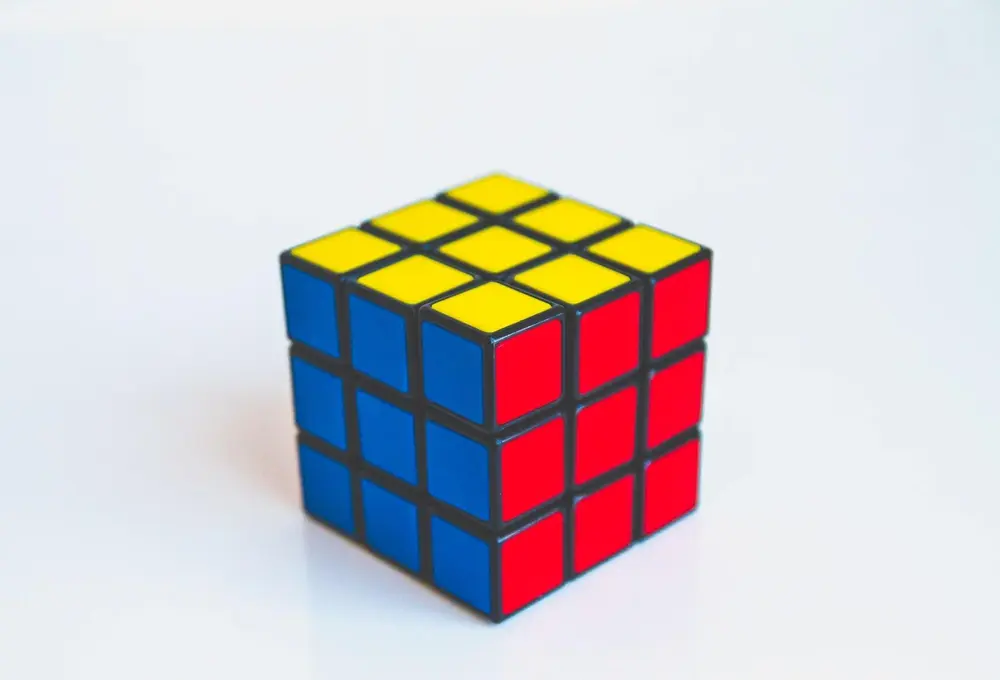# Area

Area is a two dimensional measure of space within a shape. Below, there are a variety of shapes which show how to find the area. The area of the shapes are shown as units2.

There are also several three dimensional shapes represented below. In these cases the formula shown is for the surface area of the shape (and for the cone and the cylinder it is for the curved surface area only).

Rectangle
l × w

Triangle
1/2 b h

*The area of a triangle can also be worked out by 1/2abSinC Where the sides are labelled a,b,c and the opposite angles are labelled A,B,C. This formula can be used even when the height of the triangle is not given.

Parallelogram
b × h

Trapezium
h/2 ( a + b )

Circle

r 2

Sphere

r 2

Cylinder

r h

Cone

r s

Shape and Space

Shape and Space

Shape and Space

Shape and Space

Shape and Space

Shape and Space